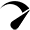# Solar Calculators

## Solar Panel Efficiency Calculator and Formula

Use our solar panel efficiency calculator to quickly calculate the efficiency of a solar panel. Also, let’s explain: Why you should care about solar panel efficiency — does it really matter? — Is it worth paying extra cash for highly efficient solar panels? How To Use Solar Efficiency Calculator? https://054d1907d090b0e00639a8b233e37dbf.safeframe.googlesyndication.com/safeframe/1-0-40/html/container.html1 – Enter solar panel maximum power output …

## Battery Runtime Calculator: How Long Does Battery Last?

How long will your battery last? find out with our easy-to-use battery runtime calculator. Calculator Assumptions Limitations Of This Calculator How To Use Our Battery Runtime Calculator? 1. Enter battery capacity in amp-hours (Ah): If the battery capacity is mentioned in watt-hours (Wh), Divide the watt-hours by battery voltage (V) to find out the battery capacity …

## Solar Battery Charge Time Calculator (12v, 24v, 48v)

Use our solar battery charge time calculator to find out how long will it take to charge a battery with solar panels. How To Use Our Solar Battery Charge Time Calculator? To use the calculator, follow these steps: 1. Enter the total solar system size in watts: If you have multiple solar panels connected together, add …

## Solar Panel Amps Calculator (Watts to Amps)

Solar Panel Amps Calculator Use our solar panel amps calculator to calculate the solar panel amps or convert solar panel watts to amps. How To Use This Calculator? Some Key Points Before You Leave We usually measure or convert the watts into amps of solar panels to figure out how much current (amps) is being …

## Battery Amp hours To Watts (Ah to Watts) Calculator

Use our battery amp-hour (Ah) to watts calculator to find out the battery capacity in watt-hours. How To Use This Calculator? How To Calculate Watts In A Battery? Watts is the unit that represents the total number of power. So to calculate watts from Ah use this formula. Watts = Amps*volts Multiplying the value of …

## Solar DC To AC Conversion Calculator & Formula (Watts, Amps)

Use our solar DC to AC conversion calculator to convert the DC (direct current) power into usable AC (alternating current) power.

Scroll to TopOptimized by Seraphinite Accelerator
Turns on site high speed to be attractive for people and search engines.